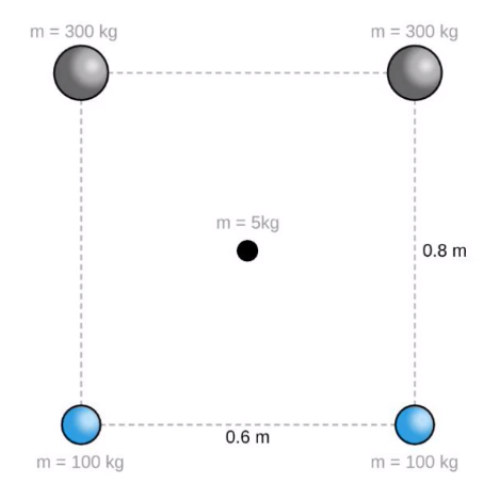Clutch Prep is now a part of Pearson
Ch 08: Centripetal Forces & GravitationWorksheetSee all chapters

# Gravitational Forces in 2D

See all sections
Sections
Uniform Circular Motion
Period and Frequency in Uniform Circular Motion
Centripetal Forces
Vertical Centripetal Forces
Flat Curves
Banked Curves
Newton's Law of Gravity
Gravitational Forces in 2D
Acceleration Due to Gravity
Satellite Motion: Intro
Satellite Motion: Speed & Period
Geosynchronous Orbits
Overview of Kepler's Laws
Kepler's First Law
Kepler's Third Law

Concept #1: Gravitational Forces in 2D

Concept #2: Using Symmetry in 2D Gravitation

Concept #3: Finding Net Forces in 2D Gravitation

Practice: Find the magnitude & direction of the net gravitational force on the center 5-kg mass in the rectangle below.